# NCERT Solution for Class 10 Mathematics Chapter 4 Quadratic Equations

 Chapter Name NCERT Solution for Class 10 Maths Chapter 4 Quadratic Equations Topics Covered Short Revision for the ChapterNCERT Exercise Solution Related Study NCERT Solution for Class 10 MathsNCERT Revision Notes for Class 10 MathsImportant Questions for Class 10 MathsMCQ for Class 10 MathsNCERT Exemplar Questions For Class 10 Maths

## Short Revision for Quadratic Equations

1. The standard form of a quadratic equation is ax2 + bx + c = 0, a ≠ 0.
2. A quadratic equation has at most two roots.
3. A real number α is said to be a root of the quadratic equation ax2 + bx + c = 0, if αa2 + bα + c = 0.
4. If α and β are two roots of a quadratic equation ax2 + bx + c = 0, then
Sum of the roots = α + β = -b/a and product of the roots
= αβ = c/a .
5. Discriminant of a quadratic equation ax2 + bx + c = 0 is given by
D = b2 – 4ac.
6. The equation ax2 + bx + c = 0 has
(i) two distinct real roots, if b2 – 4ac > 0
(ii) two equal real roots, if b2 – 4ac = 0
(iii) no real roots, if b2 – 4ac < 0.
7. The roots of a quadratic equation ax2 + bx + c = 0 are given by
8. A quadratic equation can also be solved by the method of completing te square or by factorization.
9. In the method of factorization, we generally split the linear (middle) term into two parts.
10. If α and β are the two roots of a quadratic equation, then the quadratic equation will be
k[x2 – (α + β)x + αβ] = 0
where k is a non – zero real number.
11. If the polynomial corresponding to a quadratic equation is a perfect square, then its roots are equal.
12. If each root of a quadratic equation ax2 + bx + c = 0 is zero, then b = c = 0

### NCERT Exercise Solutions

#### Exercise 4.1

1. Check whether the following are quadratic equations:
(i) (x + 1)2 = 2(x – 3)
(ii) x2 – 2x = (–2) (3 – x)
(iii) (x – 2)(x + 1) = (x – 1)(x + 3)
(iv) (x – 3)(2x +1) = x(x + 5)
(v) (2x – 1)(x – 3) = (x + 5)(x – 1)
(vi) x2 + 3x + 1 = (x – 2)2
(vii) (x + 2)3 = 2x (x2 – 1)
(viii) x3 – 4x2 – x + 1 = (x – 2)3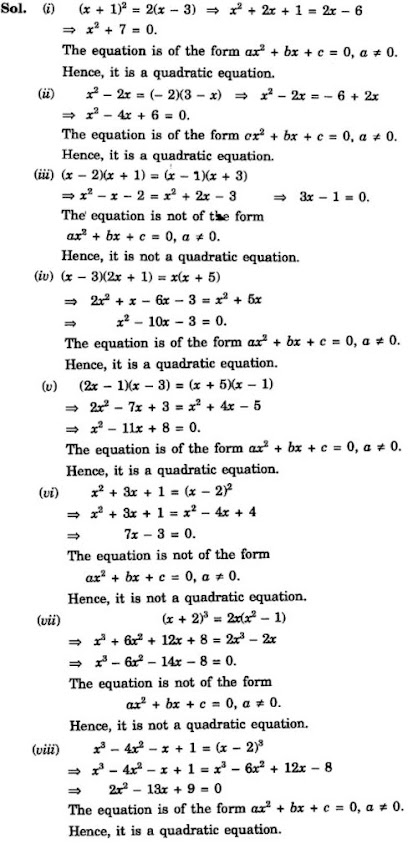2. Represent the following situations in the form of quadratic equations:
(i) The area of a rectangular plot is 528 m2. The length of the plot (in metres) is one more than twice its breadth. We need to find the length and breadth of the plot.
(ii) The product of two consecutive positive integers is 306. We need to find the integers.
(iii) Rohan’s mother is 26 years older than him. The product of their ages (in years) 3 years from now will be 360. We would like to find Rohan’s present age.
(iv) A train travels a distance of 480 km at a uniform speed. If the speed had been 8 km/h less, then it would have taken

#### Exercise 4.2

1. Find the roots of the following quadratic equations by factorisation:
(i) x2 – 3x – 10 = 0
(ii) 2x2 + x – 6 = 0
(iii) √2 x2 + 7x + 5√2 = 0
(iv) 2x2 – x +1/8 = 0
(v) 100x2 – 20x + 1 =

2. Solve the problems given in Example 1.
Represent the following situations mathematically:
(i) John and Jivanti together have 45 marbles. Both of them lost 5 marbles each, and the product of the number of marbles they now have is 124. We would like to find out how many marbles they had to start with.
(ii) A cottage industry produces a certain number of toys in a day. The cost of production of each toy (in rupees) was found to be 55 minus the number of toys produced in a day. On a particular day, the total cost of production was ` 750. We would like to find out the number of toys produced on that day.

Solution

(i) Let one of them had x marbles, then the other one had (45 - x) marbles.
∴ (x - 5)(45- x - 5) = 124
⇒ (x - 5)(40 -x) = 124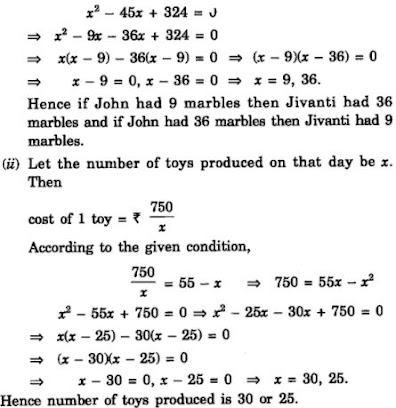3. Find two numbers whose sum is 27 and product is 182.

4. Find two consecutive positive integers, sum of whose squares is 365.

5. The altitude of a right triangle is 7 cm less than its base. If the hypotenuse is 13 cm, find the other two sides.

6. A cottage industry produces a certain number of pottery articles in a day. It was observed on a particular day that the cost of production of each article (in rupees) was 3 more than twice the number of articles produced on that day. If the total cost of production on that day was Rs.90, find the number of articles produced and the cost of each article.

#### Exercise 4.3

1. Find the roots of the following quadratic equations, if they exist, by the method of completing the square:
(i) 2x2 – 7x +3 = 0

(ii) 2x2 + x – 4 = 0

(iv) 2x2 + x + 4 = 0

2. Find the roots of the quadratic equations given in Q.1 above by applying the quadratic formula.
(i) 2x2 – 7x + 3 = 0
(ii) 2x2 + x – 4 = 0
(iii) 4x2 + 4√3x + 3 = 0
(iv) 2x2 + x + 4 = 0

3. Find the roots of the following equations:
(i) x-1/x = 3, x ≠ 0
(ii) 1/x+4 – 1/x-7 = 11/30, x = -4, 7

4. The sum of the reciprocals of Rehman’s ages, (in years) 3 years ago and 5 years from now is 1/3. Find his present age.

Solution

Let Rehman's present age = x years
Age 3 years ago = (x - 3)years
Age 5 years from now = (x + 5)years
According to given condition,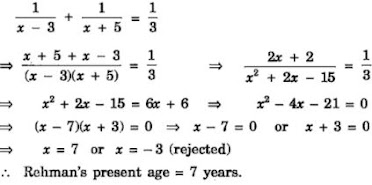5. In a class test, the sum of Shefali’s marks in Mathematics and English is 30. Had she got 2 marks more in Mathematics and 3 marks less in English, the product of their marks would have been 210. Find her marks in the two subjects.

Solution

Let marks in Mathematics = x and marks in English  = y
According to given conditions,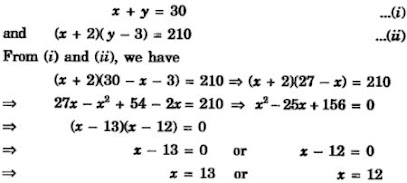When x = 13, y = 17 and when x = 12, y = 18
Hence, marks in Mathematics are 13 and in English are 17 or marks in Mathematics are 12 and in English are 18.

6. The diagonal of a rectangular field is 60 metres more than the shorter side. If the longer side is 30 metres more than the shorter side, find the sides of the field.

Solution

Let shorter side of rectangle = x m.
Then longer side = ( x +30)m and diagonal = (x + 60)m
Using Pythagoras theorem, we have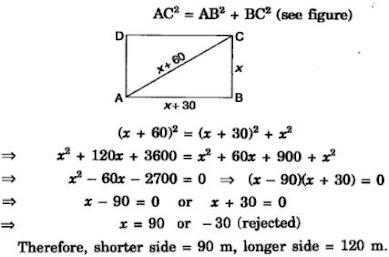7. The difference of squares of two numbers is 180. The square of the smaller number is 8 times the larger number. Find the two numbers.

8. A train travels 360 km at a uniform speed. If the speed had been 5 km/h more, it would have taken 1 hour less for the same journey. Find the speed of the train.

9. Two water taps together can fill a tank in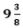hours. The tap of larger diameter takes 10 hours less than the smaller one to fill the tank separately. Find the time in which each tap can separately fill the tank.

Solution
Let time taken by smaller tap = x hours
Then time taken by larger tap = (x - 10) hours
In 1 hour, smaller tap fills 1/x of the tank
In 1 hour, larger tap fills 1/(x - 10) of the tank

10. An express train takes 1 hour less than a passenger train to travel 132 km between Mysore and Bangalore (without taking into consideration the time they stop at intermediate stations). If the average speeds of the express train is 11 km/h more than that of the passenger train, find the average speed of the two trains.

11. Sum of the areas of two squares is 468 m2. If the difference of their perimeters is 24 m, find the sides of the two squares.

Solution

Let the sides of the two squares be x m and y m respectively.
According to the given condition,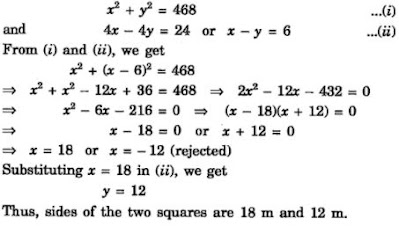#### Exercise 4.4

1. Find the nature of the roots of the following quadratic equations. If the real roots exist, find them;
(i) 2x2 – 3x + 5 = 0
(ii) 3x2 – 4√3x + 4 = 0
(iii) 2x2 – 6x + 3 = 0

2. Find the values of k for each of the following quadratic equations, so that they have two equal roots.
(i) 2x2 + kx + 3 = 0
(ii) kx (x – 2) + 6 = 0

3. Is it possible to design a rectangular mango grove whose length is twice its breadth, and the area is 800 m2? If so, find its length and breadth.

4. Is the following situation possible? If so, determine their present ages. The sum of the ages of two friends is 20 years. Four years ago, the product of their ages in years was 48.

Solution

Let present age of one friend = x years
and present age of other friend = y years
We have, x + y = 20 ...(i)
Four years ago,
Friend's age = (x - 4)years
Other friend's age = (y -4) years5. Is it possible to design a rectangular park of perimeter 80 and area 400 m2? If so find its length and breadth.